Keyah Math Study 7, Level 2

Mathematical Content : Basic algebra, solving simple equations involving kinetic energy, mass and volume

# Impact Processes: Meteor Crater, Arizona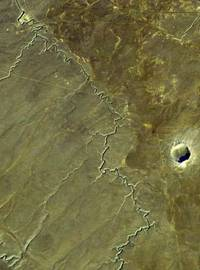Meteor Crater is a distinct impression in the relatively featureless high steppe to the west of Winslow, Arizona.  If you were, or are, seeing it for the first time, could you tell how it was formed?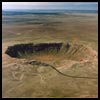It so happens that Meteor Crater is located in the vicinity of three different volcanic fields in the southwestern Colorado Plateau, so it is perhaps not surprising that some geologists initially thought it was formed by an explosive volcanic eruption.  However, no volcanic rocks occur there.  Instead, the Crater was littered with small balls of an iron-nickel alloy recognized immediately as having come from a meteoric source.  A scientific debate, which extended from the late 19th into the early 20th Century, was finally resolved in favor of an origin brought about by the impact of an iron-nickel meteorite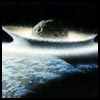The largest impacts in Earth's past would have released tremendous energy, and blasted enough material into the upper atmosphere to at least temporarily change climates.  Such catastrophes are thought to have triggered or at least contributed to mass extinctions of life on Earth, such as the extinction of the dinosaurs about 65 million years ago.  Another impact like that would pose a direct threat to the future of humanity and all other life on Earth, so it is in our best interest to understand the processes and the effects of impact cratering as thoroughly as we can!

An important question that geologists would like to answer is, “What was the size of the meteorite that formed Meteor Crater?” This is the problem that we will answer in this exercise.

Warm-up Experiment (Optional)

Warm-up Questions

1. Have you ever seen a crater that was formed by a meteor impact?

2. After looking a pictures of Meteor Crater, how wide and how deep do you think it is?

3. How big would the meteor have been to form this size crater?

4. How fast would it have been travelling?

Method of Attacking the Problem
Think about factors that would determine how “large” a crater is formed.  In part, this would depend on geological conditions specific to the impact site, such as the mechanical properties of soils and rocks.  However, one might also assume that kinetic energy, KE, of the meteorite is an important factor: the more energy delivered upon impact, the “bigger” the crater that is excavated.
There is a mathematical relationship between KE and crater diameter, we can measure the diameter of Meteor Crater and calculate the KE, and then the mass, of the meteorite.  From the mass, we can then calculate the "size" (more precisely the volume) of the meteor, because volume and mass are related by density, and we have actual fragments of the meteorite on which density has been measured. So, here’s an outline of the four-step approach we’ll take:

• Use a formula relating KE and diameter of the crater to find KE released on impact

• Use a formula relating KE and mass to find the mass of the meteorite

• Use a formula relating mass density and volume to find the volume of the meteorite

Use a formula relating volume to radius to find the diameter of the meteorite

 Important information you will need for Questions 1 and 5: The diameter of meteor crater is 1200 meters
 Question 1: How much energy was released when the meteorite formed the crater?  Read the information below for help with this question.

We will give you a formula that relates kinetic energy, KE, and diameter D of the crater. (If you want to see how this equation was obtained or find the equation yourself, click here.)

 KE = 2.499D3.250x106

The diameter D of Meteor Crater is measured in meters, and the answer for KE is in Joules.   (As a rough guide, 1 joule is the amount of energy required to lift a one kilogram object up to a height of about 10 centimetres on the surface of the Earth by the most efficient method.)
Use this equation to find the kinetic energy released from the meteorite that formed meteor crater.

 Question 2: What was the mass of the meteorite?

You can answer this question using two additional pieces of information.

 Information The equation relating mass to kinetic energy: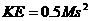where M is the mass (in kilograms, kg) of the meteorite, and s is its velocity (in meters per second). An estimate for s, the velocity of the meteor.  How fast do meteors typically travel?  The average is about 20,000 meters/sec, so use that value for s
 Question 3: How big was the meteorite, that is, what was its volume?

Again, you can answer this question by using two more bits of information and your previous answers

 Information The equation relating density, mass, and volume: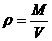where ρ (the greek letter “rho”) is the density (in kilograms per cubic meter), M is the mass, and V is the volume (in cubic meters). Fragments found at the site are iron-nickel, so the meteorite is assumed to be iron-nickel with density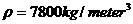.
 Question 4: What was the diameter of the meteorite?
 Notation: Assuming that the meteorite was spherical, you’ll need the formula for the volume V of a sphere: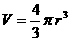, where r is the radius of the sphere (in meters).

 Question 5: How many times larger was the diameter of the crater than the diameter of the meteorite?